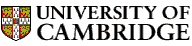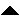Computer LaboratoryComputer Science Syllabus - Mathematics for Computation TheoryMathematics for Computation TheoryNext: Natural Language Processing Up: Michaelmas Term 2005: Part Previous: Introduction to Algorithms   Contents

## Mathematics for Computation Theory

Lecturer: Dr J.K.M. Moody

No. of lectures and practical classes: 12 + 4

This course is a prerequisite for Introduction to Security, Computation Theory, Artificial Intelligence I.

Aims

The aims of this course are to introduce the basic notation and constructs of set theory. The course will cover functions and relations, and will treat sufficient of the theory of partial orders to handle well-founded induction. The ideas are illustrated by developing the theory of finite automata and regular languages, including a proof of Kleene's Theorem.

Lectures

Part A. Discrete Mathematics

• Modelling discrete systems. Computation as a discrete process. Sets: membership, subsets, union, intersection, complement, difference. Symmetric difference. Boolean algebra. Venn diagrams. Propositions and predicates.

• Constructions on sets. Cartesian product. Disjoint union (connection with datatypes). Relations as a subset of a product. Binary relations. Functions and partial functions.

• Algebra of relations. Product and inverse relations. Composition of functions. Bijections. Function spaces. Some natural bijections between sets. Power sets. Predicates, characteristic functions. Multi-sets. n-ary predicates.

• Relations on a set. Reflexive, symmetric and transitive properties of a relation on a set. Equivalence relations. Orders, partial and total. Examples.

• Well-founded relations and the principle of induction. Well-founded relations. Their derived partial orderings. Minimal elements. Well-ordering. Examples. Mathematical induction. Well-founded induction via minimal counterexamples.

• Algebraic languages (``free algebras'') and structural induction. Languages of algebraic expressions. Free algebras. Examples. Parse trees. Well-foundedness in free algebras. Principle of structural induction for free algebras. Languages of regular expressions.

Part B. Finite Automata and Regular Languages

• Deterministic finite automata (DFA). Mechanical recognition of strings in a language. Stimulus-response behaviour, event detection. Mealey machines, Moore machines. Examples. Accepting strings via some output of a DFA. Examples.

• Using automata to recognise languages. Events/languages as sets of strings. Non-deterministic finite automata (NDFA). Proof that the language classes accepted by DFAs and NDFAs coincide.

• Algebraic operations on languages. Finite union and intersection, concatenation, and Kleene closure. Regular expressions and the languages they denote. Examples of regular languages, including recognisers.

• Regular languages are representable events. Arden's rule as a recursive definition. Its proof by induction. Extension to matrices of events. Statement of Kleene's Theorem, including an overview of the proof structure. Regular events can be represented: proof by induction on the structure of regular expressions using NDFAs.

• Representable events have regular expressions. Representable events are regular: proof by induction on the number of states using event transition matrices and Arden's rule.

• Properties of regular languages. Strings accepted by an n-state machine. The Pumping Lemma. Examples of languages that are not regular, including palindromes. Complements and intersections of regular languages are regular. Some decidable problems for regular languages. Extended regular expressions. A non-elementary problem. (Cook's tour)

Objectives

At the end of the course students should

• be familiar with the notation of set theory, and be able to use it to express simple mathematical constructs

• know how to translate between relational algebra and relational calculus

• be able to carry out simple proofs by induction, including structural induction over partially ordered (well-founded) sets

• understand how to derive a regular expression to describe the event accepted by a deterministic finite automaton

• understand how to use the Pumping Lemma to show that a given language cannot be regular

Stanat, D.F. & McAllister, D.F. (1977). Discrete mathematics in computer science. Prentice-Hall.
Hopcroft, J.E. & Ullman, J.D. (1979). Introduction to automata theory, languages and computation. Addison-Wesley.
Sudkamp, T.A. (1988). Languages and machines. Addison-Wesley. Conway, J.H. (1971). Regular algebra and finite machines. Chapman and Hall.
Forster, T.E. (2003). Logic, induction and sets. LMS Student Text 56. Cambridge University Press.
Hopcroft, J.E. & Ullman, J.D. (1974). The design and analysis of computer algorithms. Addison-Wesley.
Stone, H.S. (1973). Discrete mathematical structures and their applications. SRA.Next: Natural Language Processing Up: Michaelmas Term 2005: Part Previous: Introduction to Algorithms   Contents
Christine Northeast
Sun Sep 11 15:46:50 BST 2005© 2005 University of Cambridge Computer Laboratory Please send any comments to pagemaster@cl.cam.ac.uk Page last updated on 11-Sep-2005 at 15:57 by Christine Northeast